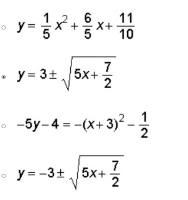# Which equation is the inverse of 5y+4 = (x+3)^2 + 1/2?

Which equation is the inverse of 5y+4 = (x+3)^2 + 1/2?